# Exploratory Analysis .pdf

### File information

Original filename: Exploratory Analysis.pdf
Title: Female Wings Exploratory Analysis
Author: Yakov Falkovich

This PDF 1.5 document has been generated by MicrosoftÂ® Word 2013, and has been sent on pdf-archive.com on 28/08/2015 at 23:10, from IP address 70.208.x.x. The current document download page has been viewed 732 times.
File size: 347 KB (5 pages).
Privacy: public file

Exploratory Analysis.pdf (PDF, 347 KB)

### Document preview

Exploratory Analysis of Female Fruit Fly Wing Sizes
Jacob Falkovich
1. Find outliers and sanitize data

Fly #1 has two wings much smaller than any in the +/+ group, probably a sick fly. THROW OUT
Fly #2 is smaller than any +/- flies, but not by as much and could conceivably be part of the main
distribution. KEEP
Fly #3 is the biggest outlier, with one normal wing and one that is tiny even compared to other outliers.
Since this is in the -/- group, throwing it out will make the variance results weaker, so conservatism also
Interestingly, there are no extra-large wing outliers, only small ones.

2. Can we just look at areas?
My naïve guess for the area of a wing would be length*(width 1 + width 2), multiplied by some constant
reflecting the wing shape. Correlations between wing area and length*(width 1 + width 2) are:
Rel
Wing
A
++
.931
+.912
-.973
Correlation across all wings: .959

B
.935
.916
.928

Correlation between length and sum of widths is .807
Interestingly, the guessed area works slightly worse for the +/- flies, implying that their shapes might be
irregular.
Bottom line: the proposed variation should affect wing growth in all dimensions, so neither length nor
width have reason to be privileged. Wing area combines the other three measurements and represents
almost all of the information contained in them.

3. Is the difference in wing area normally distributed?

Uh… more or less. At least there’s nothing that would obviously break assumptions of normality like
dichotomous peaks or huge tails.

4. So, Is there a Difference among Genotype Groups?

Visually, -/- flies (Rel=0) have lower average area and higher variance, +/- flies (Rel = 1) have higher area
and higher variance.

5. F-testing to compare two variances
+/+ and +/data: subset(females, Rel == 2)[, "Diff"] and subset(females, Rel == 1)[, "D
iff"]
F = 0.49965, num df = 42, denom df = 58, p-value = 0.009929
alternative hypothesis: true ratio of variances is less than 1
95 percent confidence interval:
0.0000000 0.8127415
sample estimates:
ratio of variances
0.4996453

+/+ and -/data: subset(females, Rel == 2)[, "Diff"] and subset(females, Rel == 0)[, "D
iff"]
F = 0.48341, num df = 42, denom df = 40, p-value = 0.01081
alternative hypothesis: true ratio of variances is less than 1
95 percent confidence interval:
0.0000000 0.8114983
sample estimates:
ratio of variances
0.483405

+/- and -/data: subset(females, Rel == 1)[, "Diff"] and subset(females, Rel == 0)[, "D
iff"]
F = 0.9675, num df = 58, denom df = 40, p-value = 0.4479
alternative hypothesis: true ratio of variances is less than 1
95 percent confidence interval:
0.00000 1.54903
sample estimates:
ratio of variances
0.9674964

There is slightly more variance among -/- (remember, we exclude an outlier with huge variance) than
among +/-, but not significantly.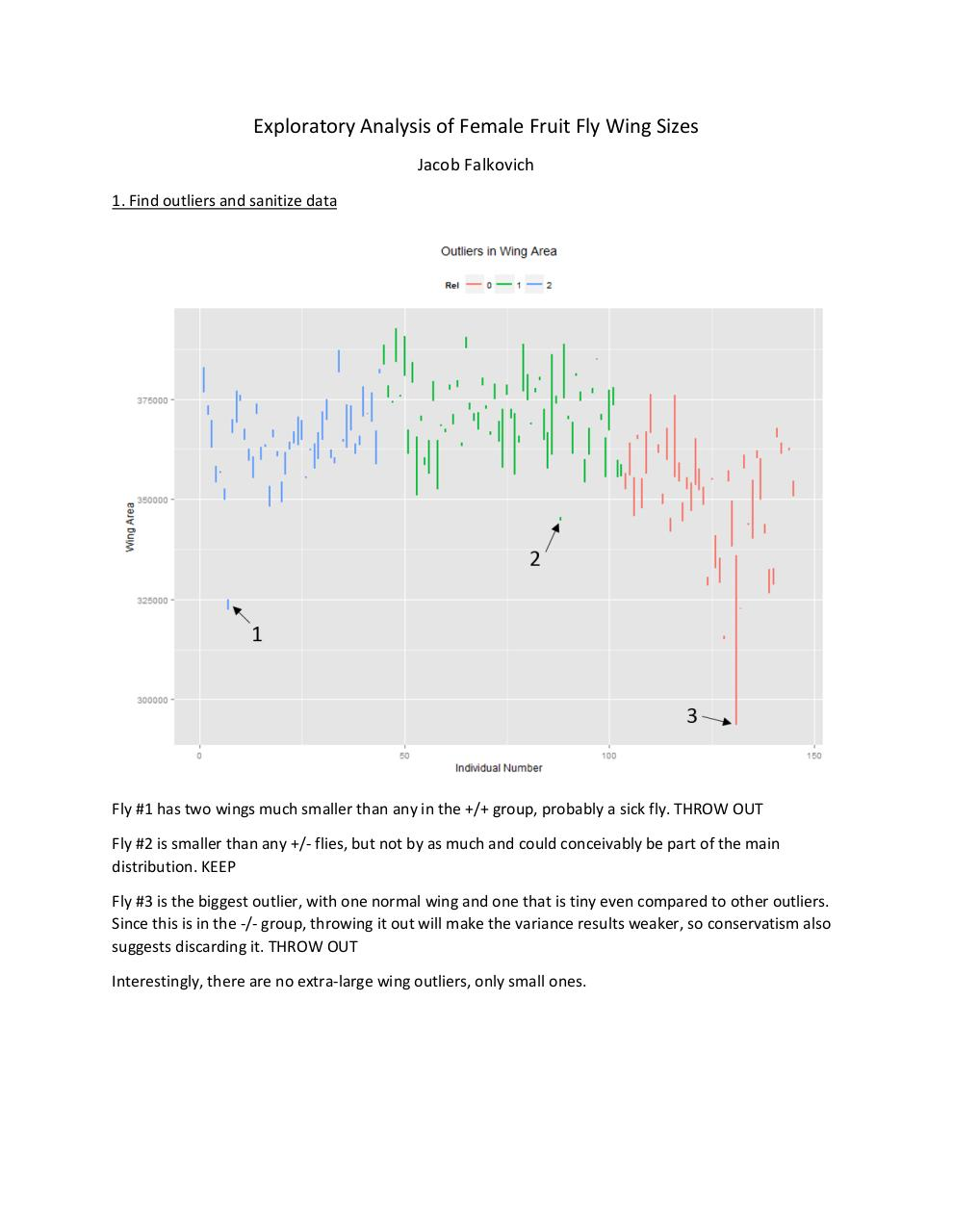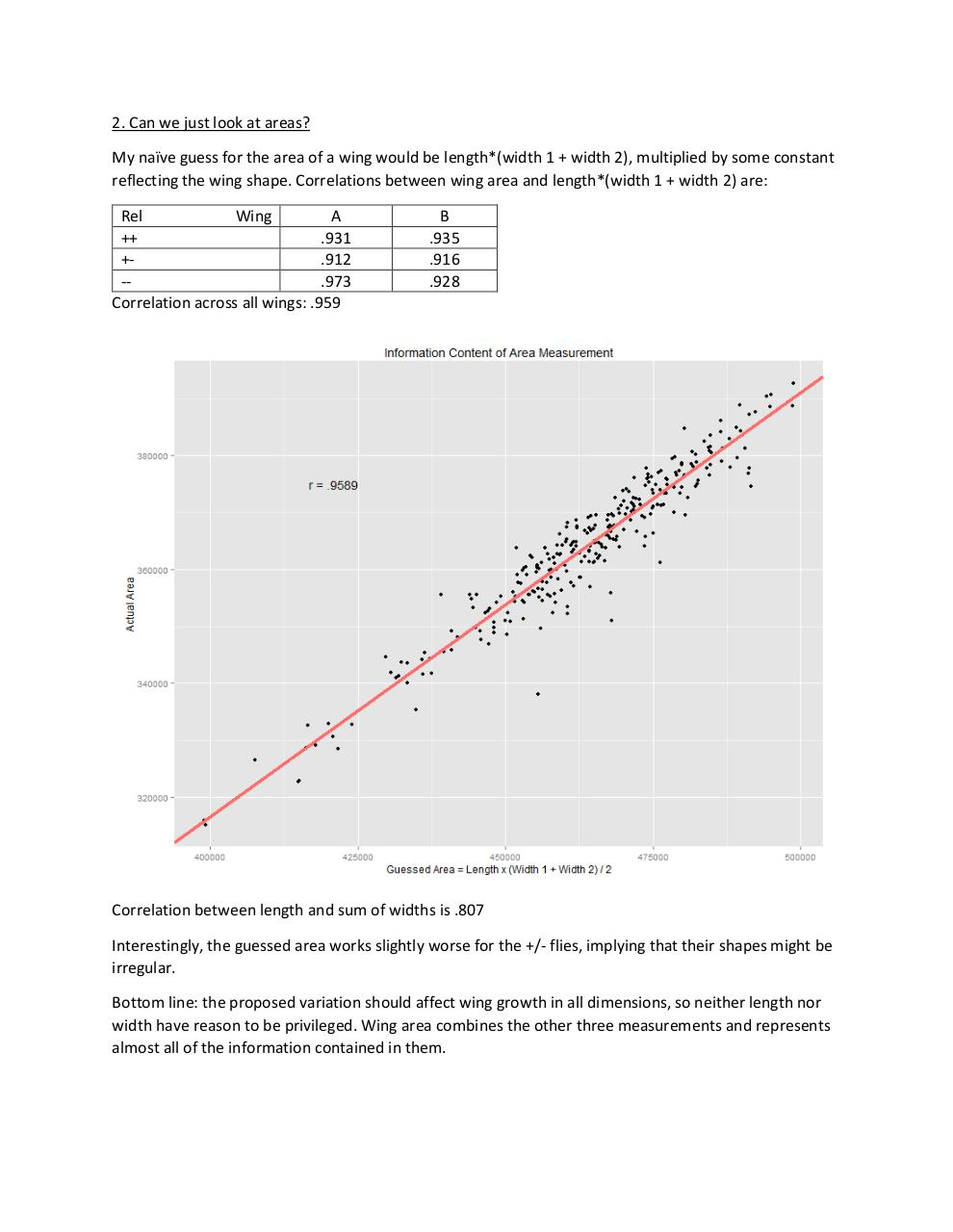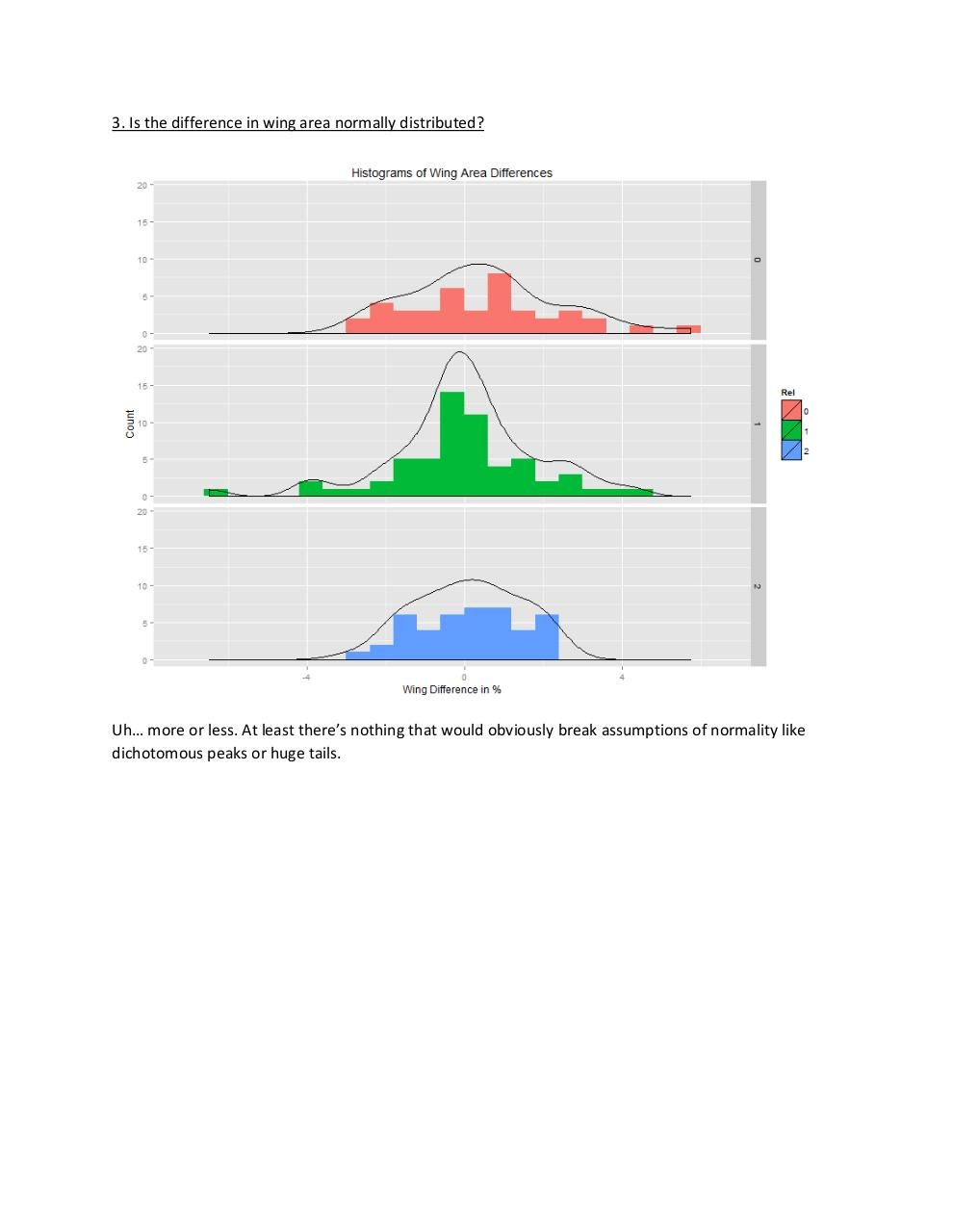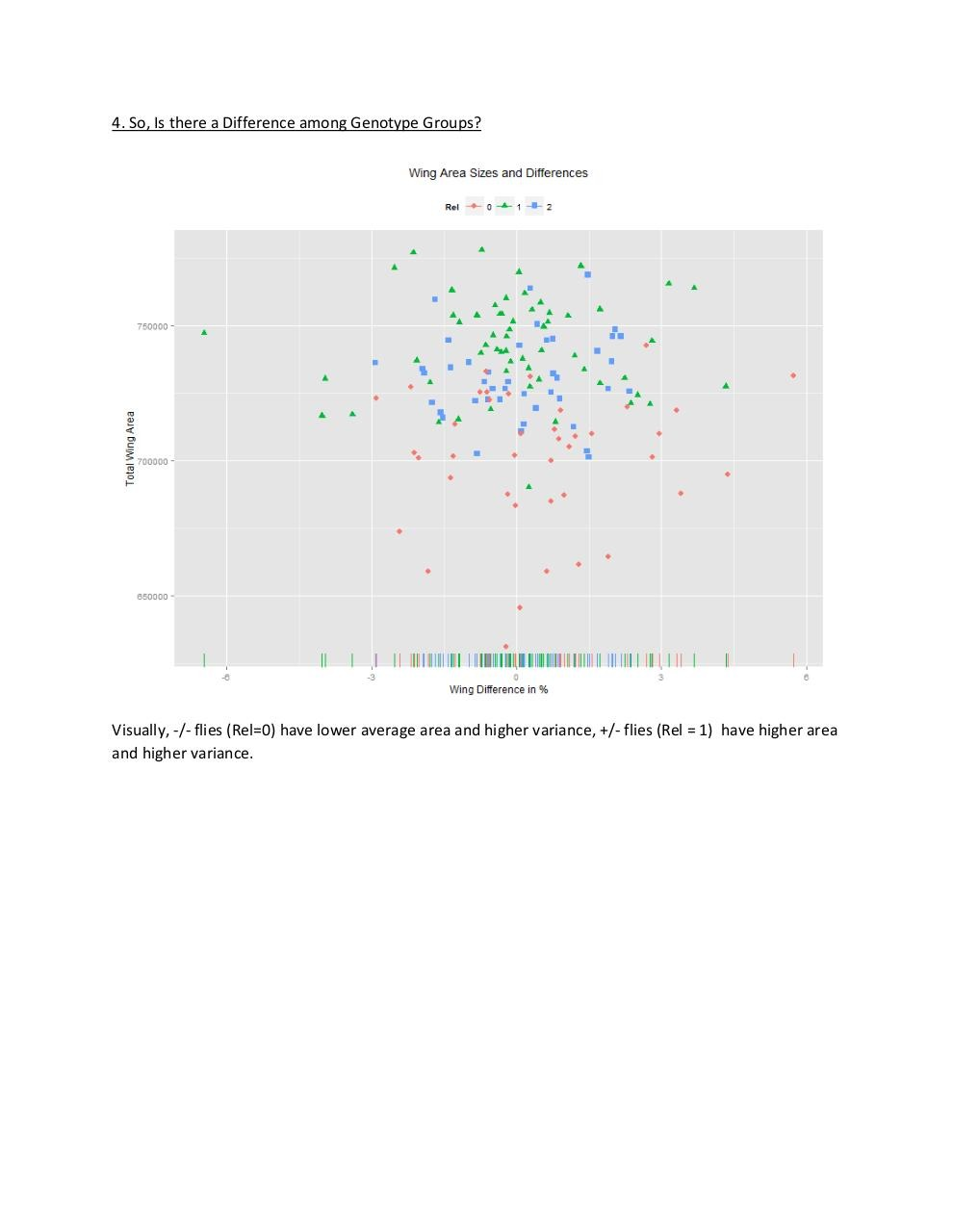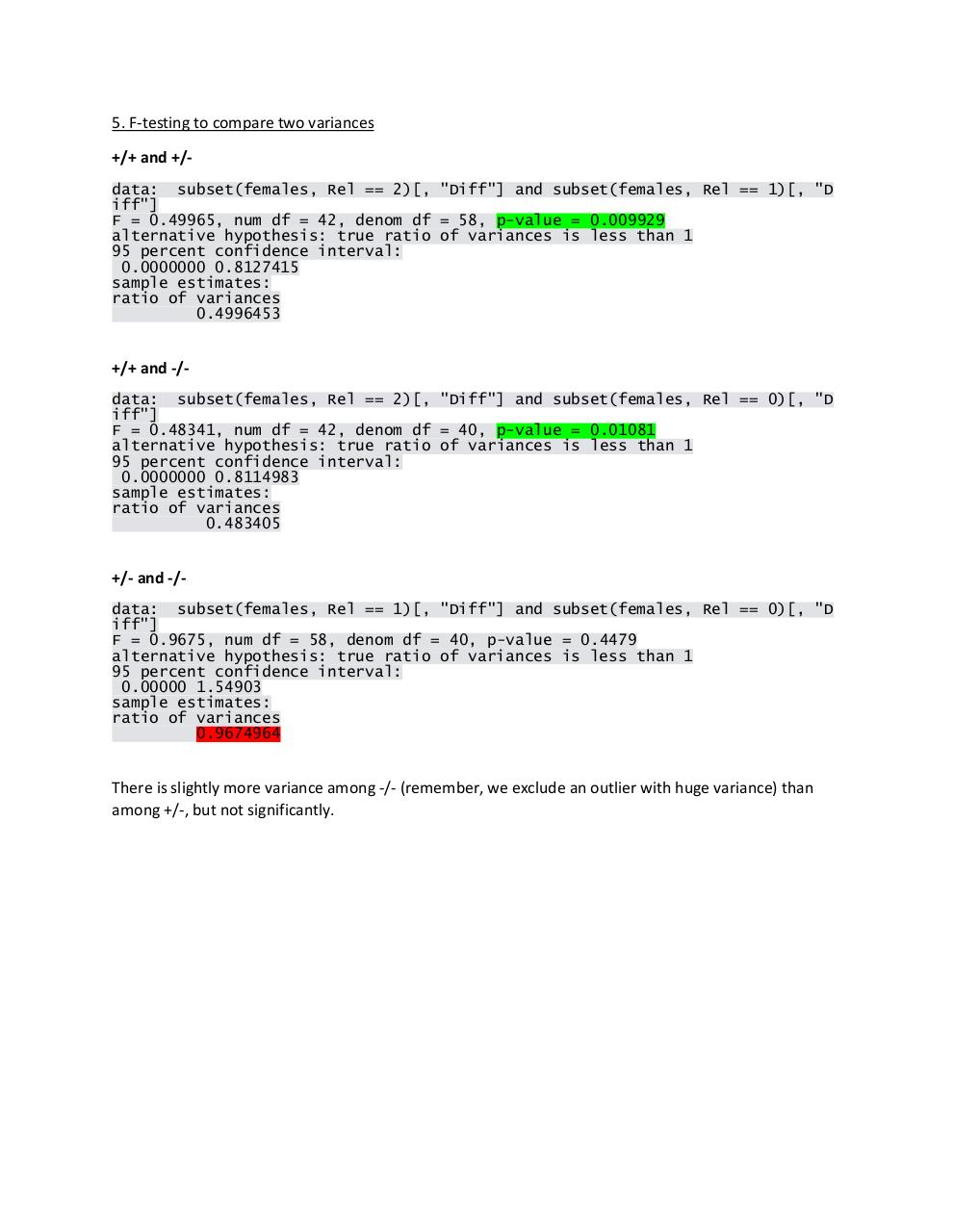#### HTML Code

Copy the following HTML code to share your document on a Website or Blog

#### QR Code### Related keywords